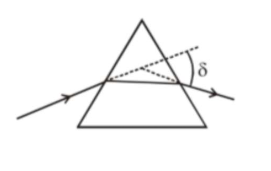# The angle of deviation through a prism is minimum when

Question:

The angle of deviation through a prism is minimum when(A) Incident ray and emergent ray are symmetric to the prism

(B) The refracted ray inside the prism becomes parallel to its base

(C) Angle of incidence is equal to that of the angle of emergence

(D) When angle of emergence is double the angle of incidence Choose the correct answer from the options given below:

1. Statements $(\mathrm{A}),(\mathrm{B})$ and $(\mathrm{C})$ are true

2. Only statement (D) is true

3. Only statements (A) and (B) are true

4. Statements (B) and (C) are true

Correct Option: 1,

Solution:

(1)

Deviation is minimum in a prism when :

$\mathrm{i}=\mathrm{e}, \mathrm{r}_{1}=\mathrm{r}_{2}$ and ray (2) is parallel to base of prism.